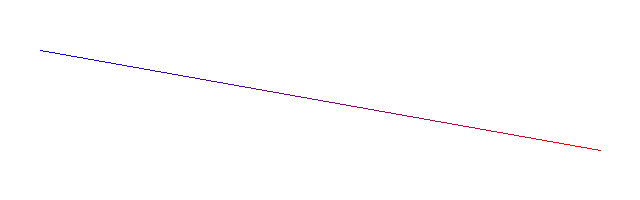# How to draw a line interpolating 2 colors with opencv

The built-in opencv `line()` drawing function allows to draw a variety of lines. Unfortunately it does not allow drawing a gradient line interpolating the colors at its start and end.

However implementing this on our own is quite easy:

``````using namespace cv;

void line2(Mat& img, const Point& start, const Point& end,
const Scalar& c1,   const Scalar& c2) {
LineIterator iter(img, start, end, LINE_8);

for (int i = 0; i < iter.count; i++, iter++) {
double alpha = double(i) / iter.count;
// note: using img.at<T>(iter.pos()) is faster, but
// then you have to deal with mat type and channel number yourself
img(Rect(iter.pos(), Size(1, 1))) = c1 * (1.0 - alpha) + c2 * alpha;
}
}``````